﻿ Optimal Design of Step Stress Partially Accelerated Life Test under Progressive Type-II Censored Data with Random Removal for Inverse Lomax DistributionPublications are Open
Access in this journal
Article Versions
Export Article
• Normal Style
• MLA Style
• APA Style
• Chicago Style
Original Article
Open Access Peer-reviewed

### Optimal Design of Step Stress Partially Accelerated Life Test under Progressive Type-II Censored Data with Random Removal for Inverse Lomax Distribution

Yasser M. Amer , Rania M. Shalabi
American Journal of Applied Mathematics and Statistics. 2019, 7(5), 171-177. DOI: 10.12691/ajams-7-5-3
Received September 12, 2019; Revised October 24, 2019; Accepted November 08, 2019

### Abstract

Step Stress-Partially Accelerated Life Test SS-PALT under Type-II progressive censoring with Binomial or uniform removal assuming Inverse Lomax distribution has been presented. A comparison between both removals is shown. The Newton-Raphson method is applied to obtain maximum likelihood estimators MLE of the parameters and the optimal stress-change time which minimizes the generalized asymptotic variance. A simulation study is performed to illustrate the statistical properties of the parameters.

### 1. Introduction

The engineers used the Partially accelerated life tests (PALTs) successfully to estimate the acceleration factor and thus extrapolating the accelerated data to normal conditions. In a PALT, items are tested at both normal and accelerated conditions. There are three Types of PALTs, these Types are step-stress, progressive-stress and constant-stress. Such testing conducted under stresses is called accelerated life test (ALT) or partially accelerated life test (PALT). In ALT, the units are put under stresses to get more failures in a short time. The main assumption in ALT is that the mathematical model relating the lifetime of the unit and the stress is known or can be assumed. In some cases, such model is neither known nor assumed. That is, ALT data cannot be extrapolated to normal use condition. So, in such cases, PALT is a more suitable test to be used to estimate the statistical model parameters.

In a SS-PALT, test unit starts at normal use condition for a specified time. If it does not fail at that time, it is putted under stress. Stress is repeatedly increased until the test unit fails or the test is terminated based on a certain censoring scheme. As indicated by 1, the step-stress method can reduce the testing time and save a lot of manpower, material sources and money. Specifically, SS-PALT should be used for reliability analysis to save time and money especially when the test units are with high reliability and the mathematical model indicated above is unknown or cannot be assumed Partially accelerated life tests (PALT) have been studied by several authors under step-stress scheme. For more details, see 2, 3, 4, 5, 6, 7, 8, 9, 10, 11, 12, 13, 14, 15, 16. It is noted that no studies have been made on the step-stress PALT under progressive censoring. In this paper, we will combine progressive censoring and step-stress PALT to develop a step-stress PALT with Progressively Type-II Censored Data using the exponential distribution as a lifetime model.

When the experimenter does not observe the lifetimes of all test units the censored sampling arises in a life test. There are two censoring schemes, Type-I censoring and Type-II censoring. Both of these two censoring schemes do not allow for units to be removed from the test at the points other than the final termination point. This allowance may be desirable when a compromise between reduced the time of experimentation and the observation of at least some extreme lifetimes is sought. Progressively censored sampling allows to the experimenter to save time and cost. The most popular one is the progressive type-II censoring scheme and it can be briefly described as follows. Suppose n identical units are put on a life testing experiment. The integer k < n is prefixed, and r1,..., rk are k prefixed non-negative integers such that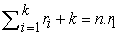of the surviving units are randomly selected and removed from the test. Similarly, at the time of the second failure, r2 units are chosen randomly from the remaining n−r1−2 units and they are removed, and so on. This experiment terminates when the mth failure occurs at time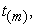the remaining surviving units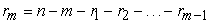are all removed from the test.

Extensive work has been done on various aspects of different progressive censoring schemes. 17 and 18 considered the progressive Type-II censoring scheme with fixed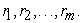But in some reliability experiments, the number of removals cannot be considered to be fixed. 19 and 20 considered progressive censoring with random (binomial) removals to estimate the unknown parameters of Weibull and Gompertz distribution using ordinary life testing. 21 used progressively Type-II censored data with binomial removals to estimate the parameters of exponential reliability model. 22 discuss step-stress partially-accelerated life test under progressive Type-II censoring with random removals.

The removals from the test are assumed to have binomial distributions. The lifetimes of the test units are considered to be exponential distributed. Recently, 23 provided Step Stress Partially Accelerated Life Test under Progressive Type-II Censored Data with Random Removal for Gompertz Distribution and the removals from the test are assumed to have binomial and uniform distributions. Also, 24 introduced the same on Frechet Distribution.

In this paper, we will use SS-PAL under progressive Type-II censoring with random removals. The removals from the test are assumed to have binomial and uniform distributions. The lifetimes of the test units are considered to be Inverse Lomax distributed. Also, we will determine the optimal stress change time which minimizes the generalized asymptotic variance of the MLE of parameters. Section 2 presents the Inverse Lomax distribution and the assumptions of the partially accelerated model. Estimation of model parameters is given in Section 3; in Section 4 and 5 simulation study results and conclusion are given.

### 2. Inverse Lomax Distribution

The Lomax or Pareto II (the shifted Pareto) distribution was proposed in 25. This distribution has found wide applications especially in analysis of the business failure life time data, income and wealth inequality, size of cities, actuarial science, medical and biological sciences, engineering, lifetime and reliability modeling. In lifetime, the Lomax model belongs to the family of decreasing failure rate in 26.

The Inverse Lomax distribution (ILD) belongs to inverted family of distributions and found to be very flexible to analyze the situation where the non-monotonicity of the failure rate has been realized in 27. If a random variable Y has Lomax distribution, then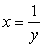has an Inverse Lomax distribution (ILD) 28. (ILD) has an application in stochastic modeling of decreasing failure rate life components. Like other distributions belonging to the family of generalized Beta distribution, the (ILD) also has application in economics and actuarial sciences 29. (ILD) was implemented on geophysical databases 30 on the sizes of land fires in the California state. 31, carried out research work regarding the statistical inference and Prediction on (ILD) through Bayesian inferences. 32 considered the (ILD) to possess the Lorenz ordering relationship between ordered statistics.

The probability density function (PDF) and the cumulative distribution function (CDF) for (ILD) respectively are as follows: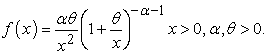(1)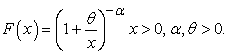(2)

### 3. Model and Assumptions

The following assumptions are used throughout the paper:

1. n identical and independent units are put on the life test.

2. The lifetime of each unit has an exponential distribution.

3. The test is terminated at the mth failure, where m is prefixed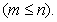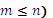4. Each of the n units is first run under normal use condition. If it does not fail or remove from the test by a pre-specified time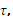. it is put under accelerated condition (stress).

5. At the ith failure a random number of the surviving units,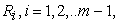are randomly selected and removed from the test. Finally, at the mth failure the remaining surviving units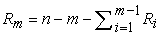are all removed from the test and the test is terminated.

6. The lifetime, say X, of a unit under SS-PALT can be rewritten as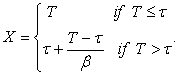In this paper, we will have assumed that, probability density function (PDF) of X is given by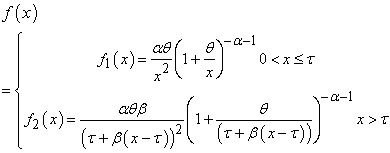(3)

In addition, the survival functions (SF) under normal and accelerate use conditions respectively is given by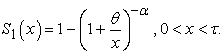(4)

And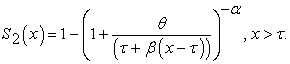(5)

### 4. Estimation of Parameters

4.1. Parameter Estimation with the Binomial Removals

The number of units removed from the test at each failure time follows a binomial distribution and any individual unit being removed is independent of others but with the same probability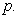that is,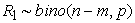and for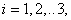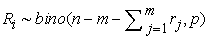and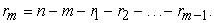Let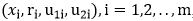, denote the observation obtained form a progressively Type-II censored sample with random removals in a SS-PALT. Here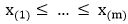. Given the pre-determined number of removals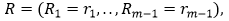the conditional likelihood function of the observations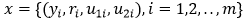takes the following form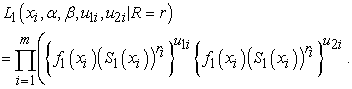(6)

From (1), (2) and (3) is inserted in (6) and simplify, we get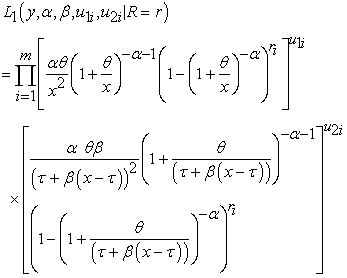(7)

Where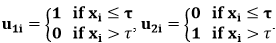And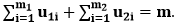The number of units removed at each failure time follows a binomial distribution such that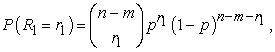and for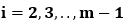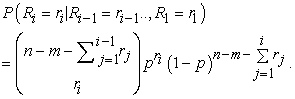(8)

At the parameter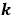is known, the likelihood of the sample of size n is given as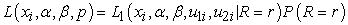(9)

Where,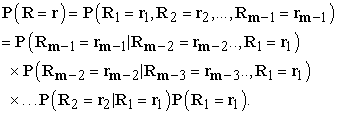That is,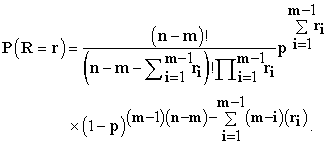(10)

The log-likelihood function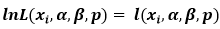can be written as follows: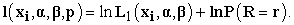(11)

First partial derivatives are derived to obtain the estimate of the parameters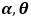and acceleration factor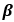. The log likelihood which is associated with (10) is given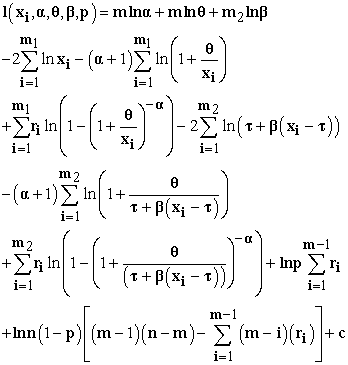Where,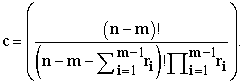The following likelihood equations are obtained by equating the partial derivatives of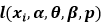with respect to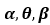and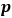to zero: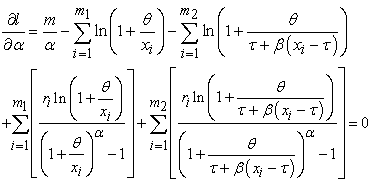(13)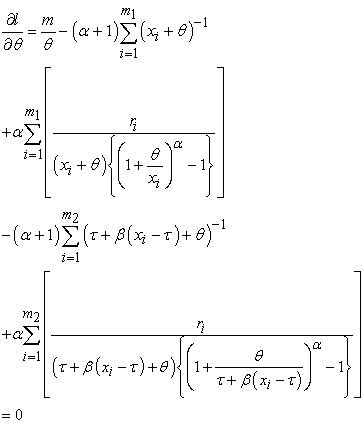(14)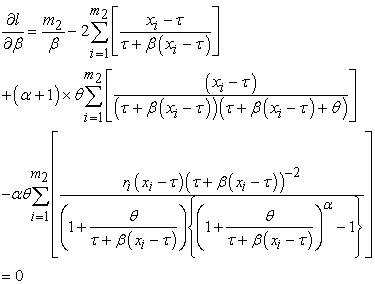(15)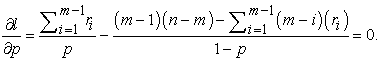(16)

From Equation (16),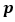is estimated as follow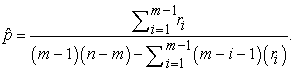(17)

There is no closed-from solution to this system of equations (13-15), so we will solve for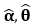and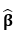iteratively, using the Newton-Raphson method, a tangent method for root finding. In our case we will estimate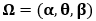iteratively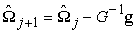(18)

where g is the vector of normal equations for which we want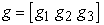with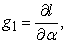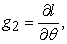and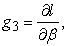and G is the matrix of second derivatives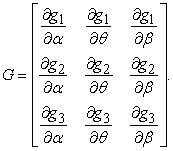(19)

The Newton-Raphson algorithm converges, as our estimates of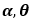and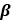change by less than a tolerated amount with each successive iteration, toand.

The bias and the root of mean squared error (RMSE) of an estimator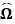of the parameter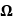, easily obtained by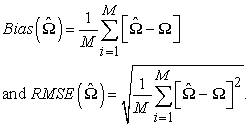(20)
4.2. Parameter Estimation with the Uniform Removals

The number of units removed from the test at each failure time follows a uniform distribution and any individual unit being removed is independent of others but with the same probability p. that is,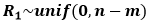and for i=1,2,..3.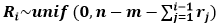and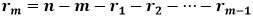.

The number of units removed at each failure time follows a uniform distribution such that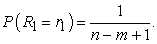(21)

And for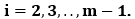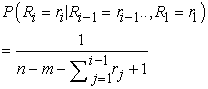where, the joint probability distribution of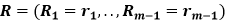and is given by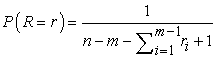(22)

where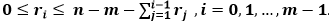.

It is clear that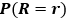does not depend on the parametersand, hence the maximum likelihood estimators can be derived directly by maximizing the equations (9) and then solving the equations (13-16).

4.3. Fisher Information Matrix

The asymptotic Fisher information matrix can be formulated as follows;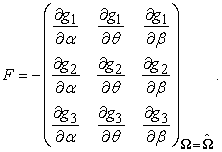(23)

In relation to the asymptotic variance-covariance matrix of the ML estimators of the parameters, it can be approximated by numerically inverting the above Fisher's information matrix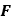.

4.4. D-optimality

In this section we explore the choice of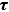in a SS-PALT with Type-II progressive censoring. We propose one selection criterion which enable one to choose the optimal value of. The proposed criterion is based on the determinant of the Fisher's information matrix. Maximizing that determinant is equivalent to minimizing the generalized asymptotic variance (GAV) of the MLE of the model parameters. The GAV is the reciprocal of the determinant of the Fisher's information matrix F, for example, see 33. That is,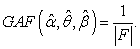(24)

So, the optimal value ofis chosen such that the determinant of the Fisher's information matrixis maximized and then the GAV is minimized. This is called the D-optimality criterion.

### 5. Simulation Study

A simulation study is performed to obtain MLEs ofand. Also, to study the properties of these estimates through the root of the mean squared errors (RMSEs),) and the confidence intervals for different sample sizes. Moreover, we will determine the optimal stress change time which minimizes the generalized asymptotic variance of the MLE of parameters. To perform the simulation study, we followed the same steps has been introduced in 24;

a) First specify the value of n and m.

b) The value of the parameters are chosen to be α=2, θ=0.5, β=2.3,τ=2, p=0.4.

c) Generate a random sample with size n and censoring size m with random removals,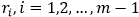from the random variable X given by (3).

d) Generate a group value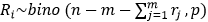and also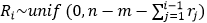where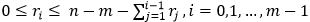and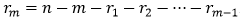.

e) For different sample sizes n= 20, 50, 80 and 100, compute the ML estimates.

The bias and the root of mean squared error (RMSE) are obtained associated with the MLE of the parameters, optimal value ofand also the Optimal GAV of the MLEs of the model parameters are obtained numerically for each sample size.

### 6. Conclusion

This paper presented the SS-PALT under Type-II progressive censoring with Binomial or uniform removals assuming (ILD). Comparison between both removals is shown. The Newton-Raphson method is applied to obtain MLE estimators of the parameters and the optimal stress-change time which minimizes the GAV.

The numerical study for obtaining the optimum plan for binomial removal is tabulated in Table 1 for different sample size and Table 2 describes uniform removal for possible values of the parameters. We derive the MLE of the parameters. Also, we compute the RMSE associated with the MLE. The above results it is easy to find that for the fixed values of the parameters, the error and optimal time decrease with increasing sample size n. Performance of testing plans and model assumptions are usually evaluated by the properties of the maximum likelihood estimates of model parameters. From the numerical results, we can conclude that both the average value ofand the average value of GAV for Type-II progressive censoring are getting close to those of complete sample with the bigger m and close faster for bigger n. Hence from the numerical result, we can conclude that estimates of binomial or uniform are stable with relatively small RMSE with increasing sample size. Therefore, the test design obtained here is a robust design and work well for binomial or uniform removal.

### References

Published with license by Science and Education Publishing, Copyright © 2019 Yasser M. Amer and Rania M. ShalabiThis work is licensed under a Creative Commons Attribution 4.0 International License. To view a copy of this license, visit http://creativecommons.org/licenses/by/4.0/